# MiniFlow - 自制玩具版 TensorFlow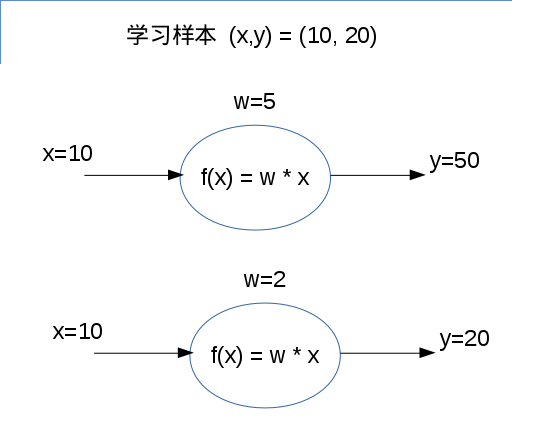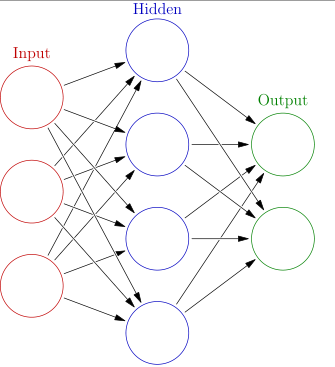By Glosser.ca - Own work, Derivative of File:Artificial neural network.svg, CC BY-SA 3.0, https://commons.wikimedia.org/w/index.php?curid=24913461

Udacity CarND-term1 Lesson 5 让我们自己去实现一个 Miniflow, 相当于一个玩具版的 Tensorflow. 通过实现 Miniflow, 可以体会到 Forward Pass 和 Backpropagation 的过程。

## 定义神经元

## 源码来自 Udacity CarND-term1 Lesson 5 ##
class Neuron:
def __init__(self, inbound_neurons=[]):
# Neurons from which this Node receives values
self.inbound_neurons = inbound_neurons
# Neurons to which this Node passes values
self.outbound_neurons = []
# A calculated value
self.value = None
# Add this node as an outbound node on its inputs.
for n in self.inbound_neurons:
n.outbound_neurons.append(self)

# These will be implemented in a subclass.
def forward(self):
"""
Forward propagation.

Compute the output value based on `inbound_neurons` and
store the result in self.value.
"""

raise NotImplemented

## 运算顺序 topological sort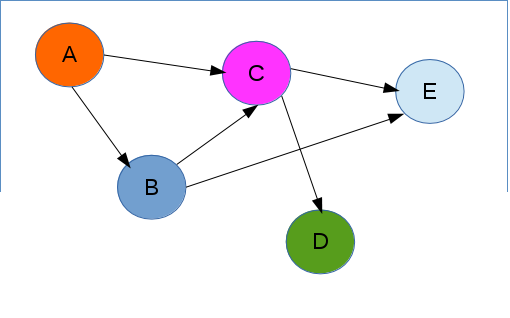## 源码来自 Udacity CarND-term1 Lesson 5 ##
def topological_sort(feed_dict):
"""
Sort generic nodes in topological order using Kahn's Algorithm.

`feed_dict`: A dictionary where the key is an `Input` node and the value is the respective value feed to that node.

Returns a list of sorted nodes.
"""

input_neurons = [n for n in feed_dict.keys()]

G = {}
neurons = [n for n in input_neurons]
while len(neurons) > 0:
n = neurons.pop(0)
if n not in G:
G[n] = {'in': set(), 'out': set()}
for m in n.outbound_neurons:
if m not in G:
G[m] = {'in': set(), 'out': set()}
neurons.append(m)

L = []
S = set(input_neurons)
while len(S) > 0:
n = S.pop()

if isinstance(n, Input):
n.value = feed_dict[n]

L.append(n)
for m in n.outbound_neurons:
G[n]['out'].remove(m)
G[m]['in'].remove(n)
# if no other incoming edges add to S
if len(G[m]['in']) == 0:
return L

## Forward Pass

Forward Pass 是把输入的数据从前往后计算，得出最终结果的过程。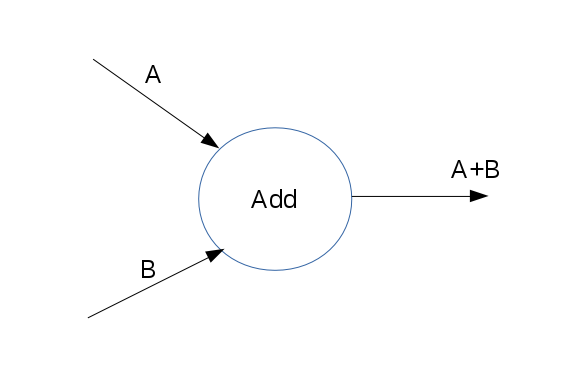## 源码来自 Udacity CarND-term1 Lesson 5 ##
def __init__(self, x, y):
# You could access `x` and `y` in forward with
# self.inbound_neurons (`x`) and self.inbound_neurons (`y`)
Neuron.__init__(self, [x, y])

def forward(self):
"""
Set the value of this neuron (`self.value`) to the sum of it's inbound_nodes.

"""

self.value = self.inbound_neurons.value + self.inbound_neurons.value

## 优化参数 - Gradient Descentcost=(20-wx)^{2}
x=5

w=2

## Backpropagation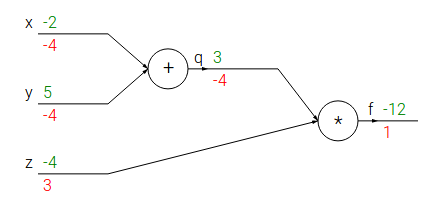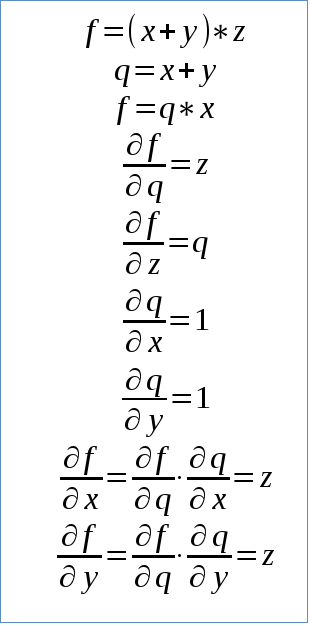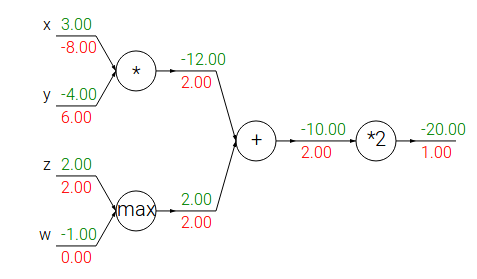## 源码来自 Udacity CarND-term1 Lesson 5 ##
class Sigmoid(Layer):
"""
Represents a layer that performs the sigmoid activation function.
"""

def __init__(self, layer):
# The base class constructor.
Layer.__init__(self, [layer])

def _sigmoid(self, x):
"""
This method is separate from `forward` because it
will be used with `backward` as well.

`x`: A numpy array-like object.
"""

return 1. / (1. + np.exp(-x))

def forward(self):
"""
Perform the sigmoid function and set the value.
"""

input_value = self.inbound_layers.value
self.value = self._sigmoid(input_value)

def backward(self):
"""
Calculates the gradient using the derivative of
the sigmoid function.
"""

# Initialize the gradients to 0.
self.gradients = {n: np.zeros_like(n.value) for n in self.inbound_layers}

# Cycle through the outputs. The gradient will change depending
# on each output, so the gradients are summed over all outputs.
for n in self.outbound_layers:
# Get the partial of the cost with respect to this layer.
"""
TODO: Your code goes here!

Set the gradients property to the gradients with respect to each input.

NOTE: See the Linear layer and MSE layer for examples.
"""

sigmoid = self.value
self.gradients[self.inbound_layers] += sigmoid * (1-sigmoid) * grad_cost